output.to from Sideway
Draft for Information Only

# Content

``` Wave Reflection inside Enclosures, 1D   Duct, 1D mode,  with two closed ends    Duct, 1D mode,  with two open ends    Duct, 1D mode,  with one end open and one end  closed ```

## Wave Reflection inside Enclosures, 1D

A sound source radiates outwardly in any direction in a free source will always encounter reflecting surfaces unless the sound source is place in an anechoic chamber where all sound energy is almost absorbed with any reflection. And in such case then only wave equation should be satisified without any boundary conditions.

Multiple reflection in an enclosure due to the wall creates standing waves with acoustic modes charactered by frequencies and mode shapes. These modes should satisfied the wave equation and boundary conditions simultaneously.

The boundary conditions are usually defined in terms of continuity between the wall motion and the particle velocity.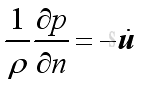The wall characteristics are usually expressed in term of impedance. A rigid wall is used to describe a wall with higher impedance while a soft wall is used to describe a wall with lower impedance.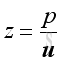### Duct, 1D mode,  with two closed ends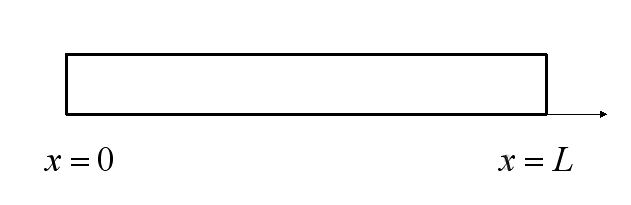The 1D wave equation is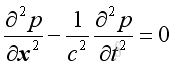Assume harmonic sound wave imply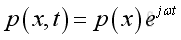Take the time differential operation, imply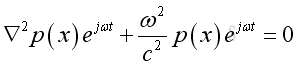And get the Helmoltz equation,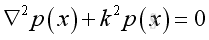Assume the solution of the equation of the form,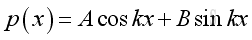Assume the two closed ends are rigid wall, the boundary conditions at two ends are with particle velocity equals to zero, implyandFrom the equation of momentum conservation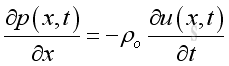At x = 0 or x=L, u=0, therefore the time derivative of u is zero also, imply: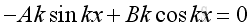From boundary condition at x=0, imply:From boundary condition at x=L, imply: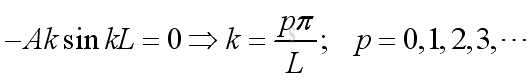Substitute k, A and B into the pressure function, imply: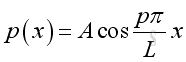and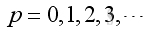Therefore the natural frequency and mode shape is: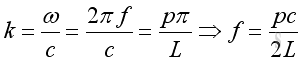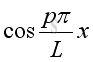and### Duct, 1D mode,  with two open endsThe 1D wave equation isAssume harmonic sound wave implyTake the time differential operation, implyAnd get the Helmoltz equation,Assume the solution of the equation of the form,With two open ends, the boundary conditions at two ends are with pressure equals to zero, imply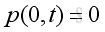and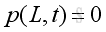From boundary condition at x=0, imply: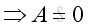From boundary condition at x=L, imply: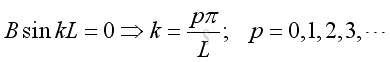Substitute k, A and B into the pressure function, and p<>0, imply: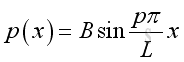and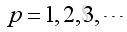Therefore the natural frequency and mode shape is: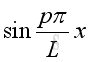and### Duct, 1D mode,  with one end open and one end  closedThe 1D wave equaton isAssume harmonic sound wave implyTake the time differential operation, implyAnd get the Helmoltz equation,Assume the solution of the equation of the form,Assume the closed end is rigid wall, the boundary conditions at closed end is with particle velocity equals to zero, implyFrom the equation of momentum conservationAt x = 0, u=0, therefore the time derivative of u is zero also, imply: o, imply:From boundary condition at x=0, imply:From boundary condition at x=L, imply: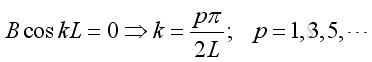Substitute k, A and B into the pressure function, imply:andTherefore the natural frequency and mode shape is: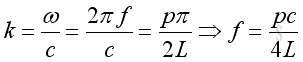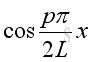andID: 101000021 Last Updated: 10/20/2010 Revision: 0 Ref:References

1. Michael P. Norton; Denis G. Karczub,, 2003, Fundamentals of Noise and Vibration Analysis for Engieer
2. G. Porges, 1977, Applied Acoustics
3. Douglas D. Reynolds, 1981, Engineering Principles of Acoustics:; Noise and Vibration Control
4. Conrad J. Hemond, 1983, Engineering Acoustics &amp; Noise Control
5. F. Fahy, 2001, Foundations of Engineering Acoustics
6. D.A. Biew; C.H. Hansen, 1996, Engineering Noise Control: Theory and PracticeHome 5

Management

HBR 3

Information

Recreation

Culture

Chinese 1097

English 337

Computer

Hardware 154

Software

Application 207

Latex 35

Manim 203

Numeric 19

Programming

Web 285

Unicode 504

HTML 65

CSS 63

SVG 9

ASP.NET 229

OS 422

Python 64

Knowledge

Mathematics

Algebra 84

Geometry 32

Calculus 67

Engineering

Mechanical

Rigid Bodies

Statics 92

Dynamics 37

Control

Acoustics 19

Natural Sciences

Electric 27